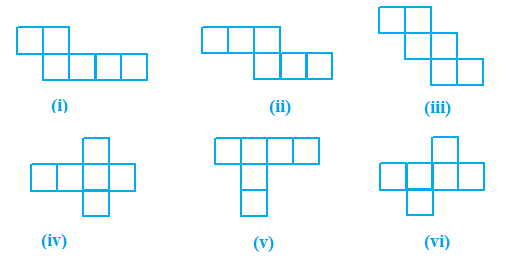# Ex.15.1 Q1 Visualising Solid Shapes Solution - NCERT Maths Class 7

Go back to  'Ex.15.1'

## Question

Identify the nets which can be used to make cubes (cut out copies of the nets and try it):Video Solution
Visualizing Solid Shapes
Ex 15.1 | Question 1

## Text Solution

What is known?

Different nets.

What is unknown?

Which nets can be used to make cube.

Reasoning:

This question is based on the concept of $$3D$$ shapes

Steps:

Figure (ii), (iii), (iv) and (vi) will make cubes. As we know a cube has $$6$$ faces, $$12$$ edges and $$8$$ vertices. So, when we will fold the nets of shapes in (ii), (iii), (iv) and (vii) we will be able to make a cube.

Learn from the best math teachers and top your exams

• Live one on one classroom and doubt clearing
• Practice worksheets in and after class for conceptual clarity
• Personalized curriculum to keep up with school